Courses

# Test: Analysis of Stress & Strain Level - 1

## 20 Questions MCQ Test Strength of Materials (SOM) | Test: Analysis of Stress & Strain Level - 1

Description
This mock test of Test: Analysis of Stress & Strain Level - 1 for Mechanical Engineering helps you for every Mechanical Engineering entrance exam. This contains 20 Multiple Choice Questions for Mechanical Engineering Test: Analysis of Stress & Strain Level - 1 (mcq) to study with solutions a complete question bank. The solved questions answers in this Test: Analysis of Stress & Strain Level - 1 quiz give you a good mix of easy questions and tough questions. Mechanical Engineering students definitely take this Test: Analysis of Stress & Strain Level - 1 exercise for a better result in the exam. You can find other Test: Analysis of Stress & Strain Level - 1 extra questions, long questions & short questions for Mechanical Engineering on EduRev as well by searching above.
QUESTION: 1

Solution:
QUESTION: 2

Solution:
QUESTION: 3

### Mohr’s circle can be used to determine, on an inclined surface

Solution:
QUESTION: 4

The extremities of any diameter on Mohr’s circle represent

Solution: Any diameter on Mohr’s circle represents states of stress on any pair of mutually perpendicular planes.

QUESTION: 5

The shear stress along the principal plane subjected to maximum principal stress is

Solution:
QUESTION: 6

In Mohr’s circle, the center of circle from y-axis is taken as

Solution:
QUESTION: 7

Maximum shearing stress planes are inclined at the following angle to the principal planes

(!) 45°

Solution:
QUESTION: 8

When a body is subjected to a direct tensile stress (σ) in the one plane. Then tangential or shear stress on oblique section of the body inclined at angle θ to the normal of the section is

Solution:
QUESTION: 9

When a square or rectangular block subjected to a shear load is in equilibrium, the shear stress in one plane is always associated with a complementary shear stress (of equal value) in the other plane at __________ to it?

Solution:
QUESTION: 10

If an element of a specimen of brittle material is subjected to shear stress, the crack propagation if occurs, will be inclined to the sides of the element at

Solution:
QUESTION: 11

The maximum shear stress from a Mohr’s circle is given by

Solution:
QUESTION: 12

Principal plane is one which carries

Solution:
QUESTION: 13

For which one of the following two-dimensional states of stress will be Mohr’s stress circle degenerate into a point?

Solution: Mohr’s circle will be a point.

=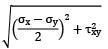∴ τxy = 0 and σx = σy = σ

QUESTION: 14

Biaxial stress system is correctly shown in

Solution: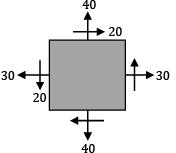Complementary shear stresses are balanced Normal stress is balanced in x and y-direction.

QUESTION: 15

If the principal stresses at a point in a strained body are σx and σyx > σy ), then the resultant stress on a plane carrying the maximum shear stress is equal to

Solution: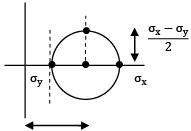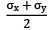σr2 =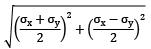=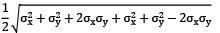=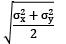QUESTION: 16

A solid cube is subjected to identical normal forces on all the faces. The ratio of volumetric strain to linear strain in any of the three axes is

Solution: For a solid cube and identical normal stresses εx = εy = εz and εv = εx + εy + εz = 3εx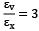QUESTION: 17

For the given stress condition σx = 2 N ⁄ mm2, σy = 0, τxy = 0, the correct Mohr’s circle is

Solution: Cannot Mohr’s stress circle.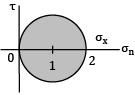QUESTION: 18

If σ1 = 600N/mm2 (tensile) and σ2 = 400 N/mm2 (Compressive), the maximum shear stress is

Solution: σ1 = 600 N/mm2 σ2 = −400 N/mm2

τmax =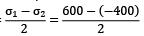= 500 N/mm2

QUESTION: 19

The principal stress induced at a point in a machine element made of steel (Syt = 460 MPa) are as follows σ1 = 210 MPa and σ2 = 145 MPa, the factor of safety according to Hencky’s theory of failure is

Solution: σ1 = 210 MPa σ2 = 145 MPa According to Hencky’s Theory (Von-Mises)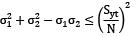(210)2 + (145)2 − 210 × 145 ≤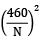N = 2.47

QUESTION: 20

A bolt is subjected to an axial load of 10 kN with a transverse shear load 4 kN and crosssection area of bolt is 250 mm2 and yield strength of bolt material is 240 MPa the factor of safety according to Guest’s theory is

Solution: σx =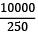= 40 MPa

τ =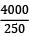= 16 MPa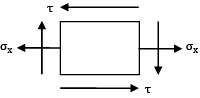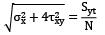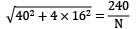N = 4.685